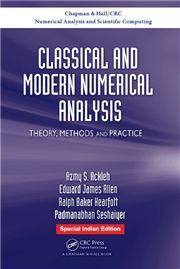# CLASSICAL AND MODERN NUMERICAL ANALYSIS: THEORY, METHODS AND PRACTICE, 1/e AZMY S. ACKLEH , EDWARD JAMES ALLEN , RALPH BAKER KEARFOTT & PADMANABHAN SESHAIYER-S.CHAND

1,195.00 1,075.00

• Mathematical Review and Computer Arithmetic
• Numerical Solution of Nonlinear Equations of One Variable
• Numerical Linear Algebra
• Approximation Theory
• Eigenvalue-Eigenvector Computation
• Numerical Differentiation and Integration
• Initial Value Problems for Ordinary Differential Equations
• Numerical Solution of Systems of Nonlinear Equations
• Optimization
• Boundary-Value Problems and Integral Equations